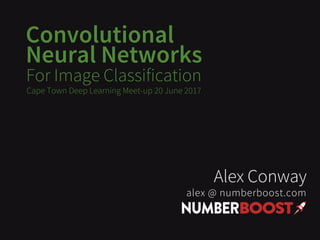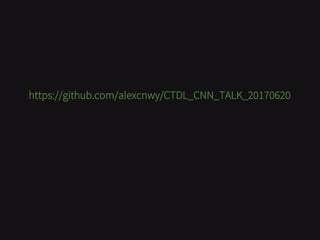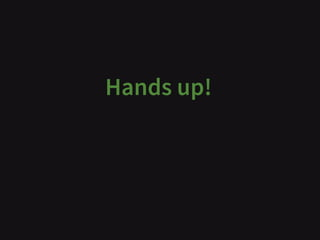Successfully reported this slideshow.

# Convolutional Neural Networks for Image Classification (Cape Town Deep Learning Meet-up 20170620)×

1 of 48
1 of 48

# Convolutional Neural Networks for Image Classification (Cape Town Deep Learning Meet-up 20170620)

Slides for my talk on:
"Convolutional Neural Networks for Image Classification"

...at the Cape Town Deep Learning Meet-up 20170620
https://www.meetup.com/Cape-Town-deep-learning/events/240485642/

Slides for my talk on:
"Convolutional Neural Networks for Image Classification"

...at the Cape Town Deep Learning Meet-up 20170620
https://www.meetup.com/Cape-Town-deep-learning/events/240485642/

### Convolutional Neural Networks for Image Classification (Cape Town Deep Learning Meet-up 20170620)

1. 1. Convolutional Neural Networks For Image Classification Alex Conway alex @ numberboost.com Cape Town Deep Learning Meet-up 20 June 2017
2. 2. https://github.com/alexcnwy/CTDL_CNN_TALK_20170620
3. 3. Hands up!
4. 4. Big Shout Outs Jeremy Howard & Rachel Thomas http://course.fast.ai Andrej Karpathy http://cs231n.github.io 4
5. 5. 1. What is a neural network? 2. What is an image? 3. What is a convolutional neural network? 4. Using a pre-trained ImageNet-winning CNN 5. Fine-tuning a CNN to solve a new problem 6. Visual similarity “latest AI technology” app 7. Practical tips 8. Image cropping 9. Image captioning 10. CNN + Word2Vec 11. Style transfer 12. Where to from here? 5
6. 6. 1. What is a neural network? 2. What is an image? 3. What is a convolutional neural network? 4. Using a pre-trained ImageNet-winning CNN 5. Fine-tuning a CNN to solve a new problem 6. Visual similarity “latest AI technology” app 7. Practical tips 8. Image cropping 9. Image captioning 10. CNN + Word2Vec 11. Style transfer 12. Where to from here? 6
7. 7. What is a Neural Network? 7
8. 8. What is a Neural Network? 8
9. 9. What is a Neural Network? 9
10. 10. http://playground.tensorflow.org
11. 11. What is a Neural Network? For much more detail, see: 1. Michael Nielson’s Neural Networks & Deep Learning free online book http://neuralnetworksanddeeplearning.com/chap1.html 2. Anrej Karpathy’s CS231n Notes http://neuralnetworksanddeeplearning.com/chap1.html 11
12. 12. What is a Neural Network? Universal Approximation theorem: http://neuralnetworksanddeeplearning.com/chap4.html 12
13. 13. What is an Image? • Pixel = 3 colour channels (R, G, B) • Pixel intensity = number in [0,255] • Image has width w and height h • Therefore image is w x h x 3 numbers 13
14. 14. What is a Convolutional Neural Network (CNN)? CNN = Neural Network + Image - with some tricks - 14
15. 15. What is a Convolutional Neural Network (CNN)? 15
16. 16. Convolutions 16 • 2-d weighted average • Element-wise multiply kernel with pixels • “learn” the kernels • http://setosa.io/ev/image-kernels/ • http://cs231n.github.io/convolutional-networks/
17. 17. Convolutions 17 “imagine taking this 3x3 matrix (“kernel”) and positioning it over a 3x3 area of an image, and let's multiply each overlapping value. Next, let's sum up these products, and let's replace the center pixel with this new value. If we slide this 3x3 matrix over the entire image, we can construct a new image by replacing each pixel in the same manner just described.”
18. 18. Convolutions 18 “...we understand that filters can be used to identify particular visual "elements" of an image, it's easy to see why they're used in deep learning for image recognition. But how do we decide which kinds of filters are the most effective? Specifically, what filters are best at capturing the necessary detail from our image to classify it? • …these filters are just matrices that we are applying to our input to achieve a desired output... therefore, given labelled input, we don't need to manually decide what filters work best at classifying our images, we can simply train a model to do so, using these filters as weights!
19. 19. Convolutions 19 “ ...for example, we can start with 8 randomly generated filters; that is 8 3x3 matrices with random elements. Given labeled inputs, we can then use stochastic gradient descent to determine what the optimal values of these filters are, and therefore we allow the neural network to learn what things are most important to detect in classifying images. “
20. 20. Convolutions 20
21. 21. Convolutions 21
22. 22. Convolutions 22
23. 23. Convolutions 23
24. 24. Max Pooling • Reduces dimensionality from one layer to next • By replacing NxN sub-area with max value • Makes network “look” at larger areas of the image at a time e.g. Instead of identifying fur, identify cat • Reduces computational load • Controls for overfitting 24
25. 25. Dropout • Form of regularization (helps prevent overfitting) • Trades ability to fit training data to help generalize to new data • Used during training (not test) • Randomly set weights in hidden layers to 0 with some probability p 25
26. 26. CNN Architectures xxx 26
27. 27. Using a Pre-Trained ImageNet-Winning CNN 27 http://image-net.org/explore
28. 28. Using a Pre-Trained ImageNet-Winning CNN 28
29. 29. Using a Pre-Trained ImageNet-Winning CNN 29 • We’ll be using “VGGNet” • Oxford Visual Geometry Group (VGG) • The runner-up in ILSVRC 2014 • Network contains 16 CONV/FC layers (deep!) • The whole VGGNet is composed of CONV layers that perform 3x3 convolutions with stride 1 and pad 1, and of POOL layers that perform 2x2 max pooling with stride 2 (and no padding) • Its main contribution was in showing that the depth of the network is a critical component for good performance. • Homogeneous architecture that only performs 3x3 convolutions and 2x2 pooling from the beginning to the end. • Easy to fine-tune
30. 30. Using a Pre-Trained ImageNet-Winning CNN 30
31. 31. Using a Pre-Trained ImageNet-Winning CNN 31 CODE TIME! https://github.com/alexcnwy/CTDL_CNN_TALK_20170620
32. 32. Fine-tuning A CNN To Solve A New Problem • Fix weights in convolutional layers (trainable=False) • Re-train final dense layer(s) 32 CODE TIME! https://github.com/alexcnwy/CTDL_CNN_TALK_20170620
33. 33. Visual Similarity “Latest AI Technology” App 33 https://memeburn.com/2017/06/spree-image-search/
34. 34. Visual Similarity “Latest AI Technology” App 34 CODE TIME! • Chop off last 2 layers • Use dense layer with 4096 activations • Compute nearest neighbours in the space of these activations https://github.com/alexcnwy/CTDL_CNN_TALK_20170620
35. 35. Practical Tips • use a GPU – AWS p2 instances not that expensive – much faster • use “adam” / different optimizers SGD variants – http://sebastianruder.com/content/images/2016/09/saddle_point_evaluation_optimizers.gif • look at nvidia-smi • when overfitting - try lower dropout and train longer • when underfitting, try: 1. Add more data 2. Use data augmentation – flipping – slightly changing hues – stretching – shearing – rotation 3. Use more complicated architecture (Resnets, Inception, etc) 35
36. 36. Pseudo Labelling 36
37. 37. Pseudo Labelling 37
38. 38. Pseudo Labelling 38
39. 39. Image Cropping Label the bounding boxes Learn to predict them Just extra input to CNN 39
40. 40. Image Captioning XXX 40
41. 41. CNN + Word2Vec 41
42. 42. CNN + Word2Vec 42
43. 43. CNN + Word2Vec 43
44. 44. CNN + Word2Vec 44
45. 45. CNN + Word2Vec 45 • Learn the word2vec vectors for each ImangeNet noun
46. 46. Style Transfer • http://blog.romanofoti.com/style_transfer/ • https://github.com/junyanz/CycleGAN 46
47. 47. Where to From Here? • Clone the repo and train your own model • Do the fast.ai course • Read the cs231n notes • Read http://colah.github.io/posts • Email me questions /ideas :) alex@numberboost.com 47
48. 48. THANKS! https://github.com/alexcnwy/CTDL_CNN_TALK_20170620 Alex Conway alex @ numberboost.com